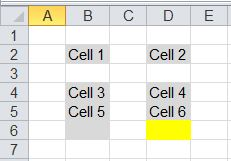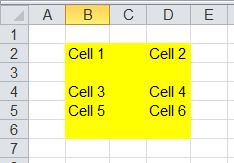# Excel Range

On this page you will find some special ranges and how to define them in AutoIt.
As input file I will use the following Excel workbook. All cells which have a value now or had a value before (means: all cells which had been touched by the user or a script) are displayed in grey.All examples show cells of the selected range in yellow.

## Active cell

The currently selected cell.

```\$oRange = \$oExcel.ActiveCell
```

## Current Region

The current region is a range bounded by any combination of blank rows and blank columns.
Note that cell B6 is not part of the region. The cell has been touched before but now is blank and hence bounds the region.

```\$oRange = \$oWorkBook.Activesheet.Range("B4").CurrentRegion
```

## Empty Cells

Returns a range object including all empty cells in the specified range.

```\$oRange = \$oWorkbook.ActiveSheet.UsedRange.SpecialCells(\$xlCellTypeBlanks)
```

## Entire Row / Entire Column

Returns a range object including the entire row(s) of the specified range.
Use method "EntireColumn" to select the columns of the specified range.

```\$oRange = \$oWorkBook.Activesheet.Range("B2").EntireRow
```

## Last Cell

Returns a range object including the last used cell (bottom right) in the used range.
External Link: Excel Ranges: Finding the Last Cell in a Range

```\$oRange = \$oWorkbook.ActiveSheet.UsedRange.SpecialCells(\$xlCellTypeLastCell)
```Returns a range object including the last used cell in column "B".
NB: If the script moved up to row 1 you need to check if the cell is really the last used cell or just the top of the range.

```Local Const \$xlUp = -4162
With \$oWorkbook.ActiveSheet ; process active sheet
; Returns a Range that contains the last used cells
\$oRangeLast = .UsedRange.SpecialCells(\$xlCellTypeLastCell)
; Get the row count of the range starting in row/column 1 and ending at the last used row/column
\$iRowCount = .Range(.Cells(1, 1), .Cells(\$oRangeLast.Row, \$oRangeLast.Column)).Rows.Count
; Start in the row following the last used row and move up to the first used cell in column "B" and grab this row number
\$iLastCell = .Cells(\$iRowCount + 1, "B").End(\$xlUp).Row
ConsoleWrite("Last used cell in column B: " & \$iLastCell & @CRLF)
EndWith
```

## Used Range

Returns a range object including all cells which have a value now or had a value before (means: all cells which had been touched by the user or a script).

Note that the range in the following example does not contain row 1 and column 1!

```\$oRange = \$oWorkBook.Activesheet.UsedRange
```Returns the address of the upper left cell of the used range. In this examle: B2

```\$sUpperLeftCell = \$oWorkbook.ActiveSheet.UsedRange.Cells(1, 1).Address
```

```With \$oWorkbook.ActiveSheet
```\$oRange = \$oExcel.Selection